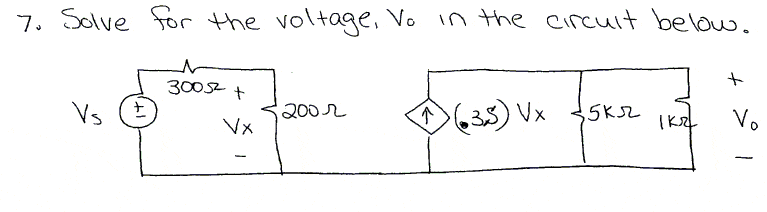# Solving for Vo in terms of Vs/Vx

http://imgur.com/xe52P30

2. Vx= Vs(R1/R1+R2), V=IR

3. I combined the 1k Ω and 5k Ω resistors then used voltage division to find Vx. I used Vx to find Vo which I found to be (5/18)Vx. I dont know where I can do KCL or KVL to solve for Vx.

Last edited:

## Answers and Replies

gneill
Mentor2. Vx= Vs(R1/R1+R2), V=IR

3. I combined the 1k Ω and 5k Ω resistors then used voltage division to find Vx. I used Vx to find Vo which I found to be (5/18)Vx. I dont know where I can do KCL or KVL to solve for Vx.

You used voltage division to find Vx. What did you get?

Can you show your work for finding Vo in terms of Vx? The expression you gave doesn't look right to me.

#### Attachments

Doing voltage division I found Vx = (2/5)Vs. After combining the two resistors on the right side and doing ohm's law I found that Vo = (5/6)(1/.3)Vx = (mistake) is 5/1.8=2.8Vx.

gneill
Mentor
Check your value for the combined resistors. Pay attention to the units used. Also take a look at your current expression; why do you divide by 0.3S? The current is specified to be 0.3S*Vx.

•1 person
Check your value for the combined resistors. Pay attention to the units used. Also take a look at your current expression; why do you divide by 0.3S? The current is specified to be 0.3S*Vx.

Does .3S not mean .3 Siemens = 1/Resistance ?

gneill
Mentor
Does .3S not mean .3 Siemens = 1/Resistance ?

Yes. And Volts x Siemens = Current. That's a controlled current source.

Yes. And Volts x Siemens = Current. That's a controlled current source.

Ok, so then Vo=.25Vx?, I also have Vx=.4Vs

gneill
Mentor
Ok, so then Vo=.25Vx?, I also have Vx=.4Vs

Again, what are the units for the two resistors in parallel? How many ohms does their parallel combination make? Your expression for Vx looks okay.

Again, what are the units for the two resistors in parallel? How many ohms does their parallel combination make? Your expression for Vx looks okay.

They are in kiloohms, their parallel combination makes (5*1)/(5+1) = 5/6 kΩ, 833Ω

gneill
Mentor
They are in kiloohms, their parallel combination makes (5*1)/(5+1) = 5/6 kΩ, 833Ω

Right. Or, if you want to retain accuracy, call it 5000/6 = 2500/3 Ohms. So multiplying your 0.3Vx by 2500/3 gives...

Vo=250Ω*Vx, so now I have Vx = .4Vs and Vo = 250Vx. I could substituite Vx into Vo, but I do not have a value for Vs.

gneill
Mentor
Vo=250Ω*Vx, so now I have Vx = .4Vs and Vo = 250Vx. I could substituite Vx into Vo, but I do not have a value for Vs.

Nor do you need one... you are looking for Vo in terms of Vs, so a symbolic solution is fine.

•1 person
Nor do you need one... you are looking for Vo in terms of Vs, so a symbolic solution is fine.

Oh well then, thanks for the help.